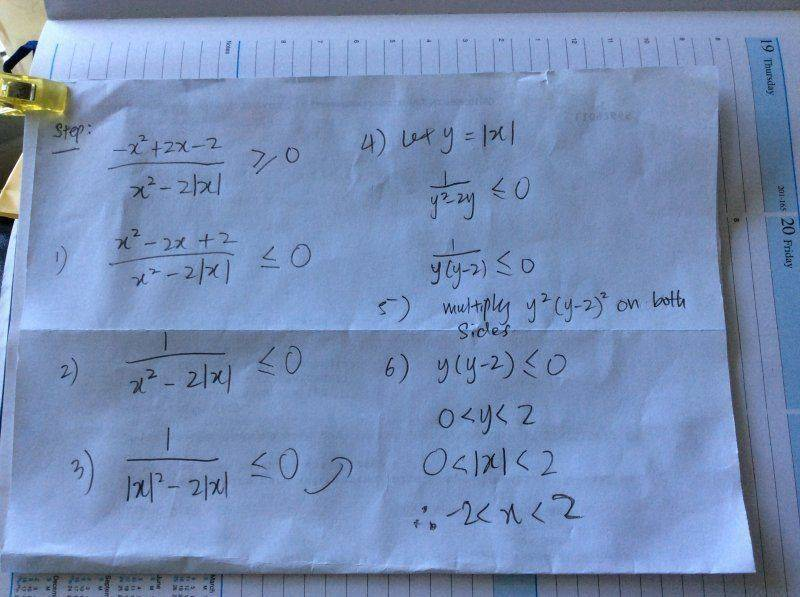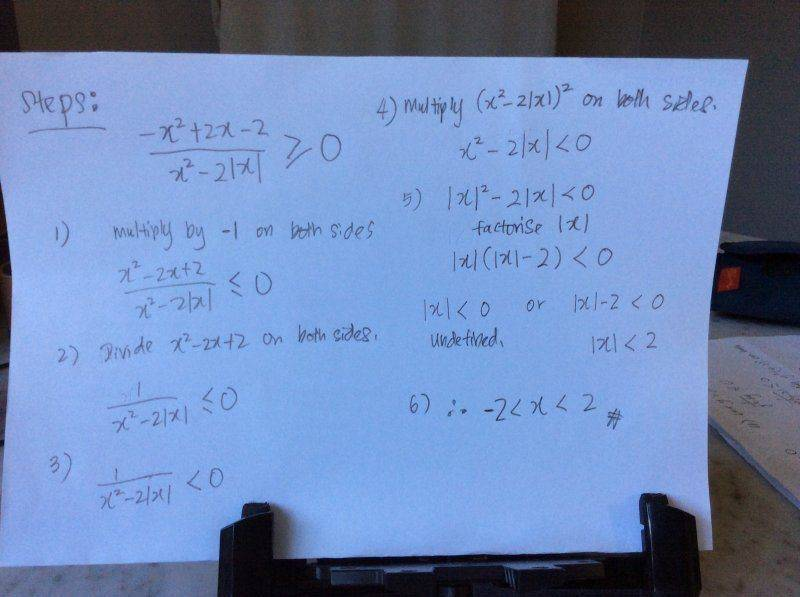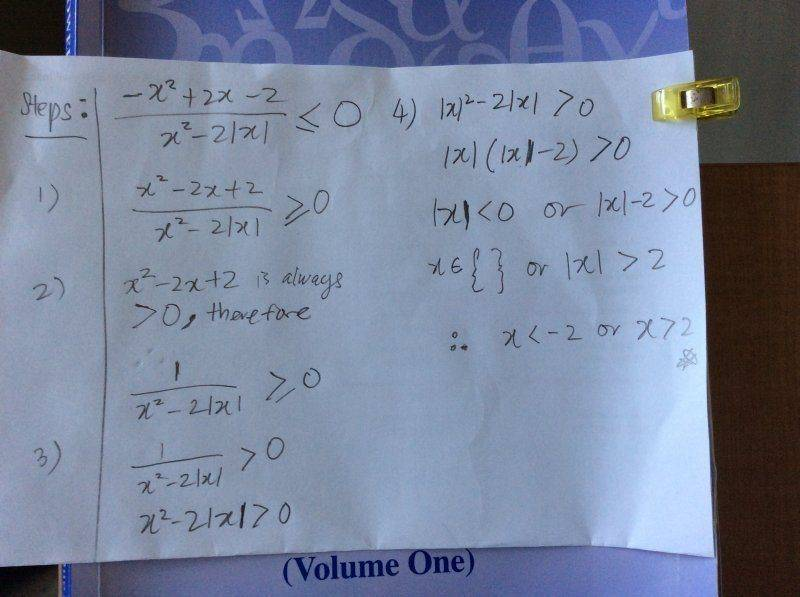# Quadratic inequality involving Modulus Function

LiHJ

## Homework Statement

Dear Mentors and PF helpers,

I saw this question on a book but couldn't understand one part of it.

Here the question:
Solve the following inequality
I copied the solution as below

## Homework Equations## The Attempt at a Solution

I don't understand why the numerator in step (1) can become 1 in step (2).
Can someone explain the concept behind.

Homework Helper

## Homework Statement

3. The Attempt at a Solution [/B]
I don't understand why the numerator in step (1) can become 1 in step (2).
Can someone explain the concept behind.
The numerator at 1) is (x-1)2, EdiT: (x-1)2 + 1, it can not be negative. You can divide by it, and the inequality holds.

Last edited:
PWiz
The numerator at 1) is (x-1)2, it can not be negative. You can divide by it, and the inequality holds.
I don't think ##x^2 -2x +2## factorizes to ##(x-1)^2## , and since the discriminant of the numerator in step 1 is negative, there will be no real solution to it. Hence, the numerator can be taken out of the equation by cross multiplication, and this leads to step 2. (I think so)

Homework Helper
I don't think ##x^2 -2x +2## factorizes to ##(x-1)^2## , and since the discriminant of the numerator in step 1 is negative, there will be no real solution to it. Hence, the numerator can be taken out of the equation by cross multiplication, and this leads to step 2. (I think so)
You are right, I made a mistake when typing in. The numerator is (x-1)2+1, can not be negative.
If the numerator could not be zero, (had no roots) it could be entire negative. Dividing by it would change the inequality then . 2) follows from 1) only when the numerator is always positive.

PWiz
Just curious - is step 4) unnecessary? Can step 3 not be simplified to ##x^2-2 |x|## < 0 (Since 1/a can never be equal to 0)?

Homework Helper
Just curious - is step 4) unnecessary? Can step 3 not be simplified to ##x^2-2 |x|## < 0 (Since 1/a can never be equal to 0)?[/QUOTE ]

You are right . From ##\frac {1}{x^2-|x|}<0## (equality is not allowed) x^2-|x|<0 follows. Step 4) is not needed. So |x|(|x|-2)<0 --> |x|<2.

LiHJ
Dear ehild and PWiz,

I have corrected it.There's one more thing that I need clarification is that on ehild 2nd post, states that if the numerator could not be zero, it coluld be entire negative.

What this means.
I know that if we multiply or divide negatives, the sign of inequality would change.

Homework Helper
The numerator is always positive. So we need to drop the equality.

You can not divide a non-zero number by zero, so the denominator can not be zero, either.

You can divide the inequality by the positive numerator, so the inequality simplifies to the form 1/A<0. Step 4) is not necessary. You know that 1/A is negative only when A is negative. But you may do 4), it is correct. And the whole solution is correct, but |x|<0 is impossible,so exlude x=0 from he solution.

When I said that if the numerator can not be zero, it can be either entirely positive or entirely negative, it was answer to PWiz.

Last edited:
PWiz
As ehild has correctly pointed out, if the left side of the equation cannot equal 0, then the inequality can be simplified to being less than 0 as you've done is step 3(the expression on the LHS is -ve). For step 5, you can avoid the inequality |x|<0 by dividing both sides by |x| right at the beginning of step 5, and the inequality will be preserved (since |x| is always positive). So you get |x|-2 <0 (only), and then you can get the proper solution, just as how you've done in the last step.

Last edited:
Homework Helper
X=0 has to be excluded from the solution.

LiHJ
Thank you ehild and PWiz,

Now I changed the question the other way round, it has to be less than or equal to zero.Homework Helper
A product of two factors can be positive only when either both factors are positive or both of them are negative. One of the factors is |x|. It is never negative, so the other factor |x|-2 is also positive.